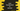# Java program to convert a Hexadecimal value to Decimal## Java program to convert a Hexadecimal value to Decimal:

In this post, we will learn how to convert a Hexadecimal value to decimal in Java. We will learn different ways to do the conversion. The hexadecimal value will be stored in a string and the converted decimal value will be an integer.

Hexadecimal and Decimal, both are different number systems. The hexadecimal system uses 16 as its base and the decimal system uses 10 as its base. For decimal number system, we need to use 0, 1, 2, 3, 4, 5, 6, 7, 8, and 9 as the numerals or digits to represent a number.

In hexadecimal system, we have to use 0, 1, 2, 3, 4, 5, 6, 7, 8, 9, A, B, C, D, E, and F to represent a number. We can also use lowercase characters i.e. a, b, c, d, e, and f to represent a hexadecimal number.

### Algorithm to convert a hexadecimal number to decimal:

To convert a hexadecimal number to decimal, we have to iterate through the digits of the hexadecimal number one by one from end to start. The following algorithm we can use to convert a hexadecimal number to decimal.

• Iterate through the digits of the hexadecimal number from the rightmost digit to the leftmost digit.
• Multiply the decimal equivalent of the hexadecimal value with 16^n where n starts from 0. For example if it is a or A, we have to take 10 to multiply.
• Add the multiplication value to a final sum value. This value will be the required decimal value.

### Method 1: Java program to convert a hexadecimal number to decimal by iterating through the digits of the number:

Let’s use the above algorithm to convert a hexadecimal value to decimal:

``````import java.util.Scanner;

class Main {

static int hexaToDecimal(char c) {
return switch (c) {
case '0' -> 0;
case '1' -> 1;
case '2' -> 2;
case '3' -> 3;
case '4' -> 4;
case '5' -> 5;
case '6' -> 6;
case '7' -> 7;
case '8' -> 8;
case '9' -> 9;
case 'A', 'a' -> 10;
case 'B', 'b' -> 11;
case 'C', 'c' -> 12;
case 'D', 'd' -> 13;
case 'E', 'e' -> 14;
case 'F', 'f' -> 15;
default -> -1;
};
}

static int hexaToDecimal(String str) {
int decimal = 0;
int multiplier = 1;
for (int i = str.length() - 1; i >= 0; i--) {
decimal += hexaToDecimal(str.charAt(i)) * multiplier;
multiplier *= 16;
}
return decimal;
}

public static void main(String[] args) {
Scanner sc = new Scanner(System.in);

}
}``````

In this program,

• hexaDecimal is a String variable to hold the user input hexadecimal value. sc is a Scanner object to read the user input value.
• It calls the hexaToDecimal method to get the decimal value.

• The hexaToDecimal method has two overloading methods. It can take either a string or character as the parameter and it returns the integer value.
• It iterates through the characters of the hexadecimal value from end to start and calculates the decimal by using the algorithm we discussed before. The calculated value is stored in the decimal variable.
• It returns the decimal at the end of the function.

You will get output as like below:

``````Enter the hexadecimal value:
Decimal value: 4525

Decimal value: 4525``````

### Method 2: By using Integer.parseInt:

The parseInt method defined in the Integer class can be used to parse a string argument. We can pass a string and a radix value and it will return the integer value of the parsed string. Each character of the string should be a valid digit for that specific radix.

This method is defined as like below:

``public static int parseInt(String s, int radix)``

Here, the first parameter s is the string to parse and the second parameter radix is the radix to use for parsing. It uses the radix to parse the string value.

It might throw a NumberFormatException if:

• The string is null or empty string or an invalid string.

Otherwise, it will return the parsed integer value.

Let’s rewrite the above program using Integer.parseInt:

``````import java.util.Scanner;

class Main {

public static void main(String[] args) {